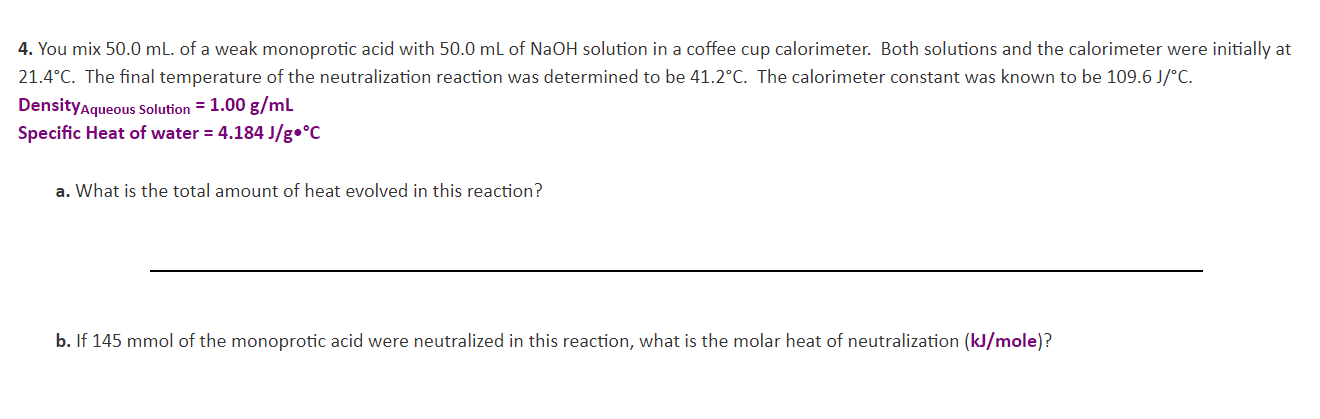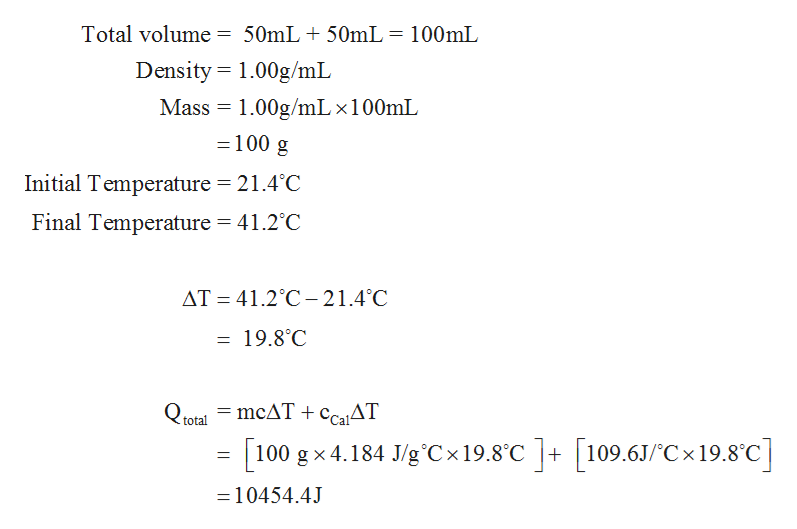# 4. You mix 50.0 mL. of a weak monoprotic acid with 50.0 mL of NaOH solution in a coffee cup calorimeter. Both solutions and the calorimeter were initially at21.4°C. The final temperature of the neutralization reaction was determined to be 41.2°C. The calorimeter constant was known to be 109.6 J/°C.DensityAqueous Solution = 1.00 g/mLSpecific Heat of water 4.184 J/g.°Ca. What is the total amount of heat evolved in this reaction?b. If 145 mmol of the monoprotic acid were neutralized in this reaction, what is the molar heat of neutralization (kJ/mole)?

Question
27 views

Please solve for a and bhelp_outlineImage Transcriptionclose4. You mix 50.0 mL. of a weak monoprotic acid with 50.0 mL of NaOH solution in a coffee cup calorimeter. Both solutions and the calorimeter were initially at 21.4°C. The final temperature of the neutralization reaction was determined to be 41.2°C. The calorimeter constant was known to be 109.6 J/°C. DensityAqueous Solution = 1.00 g/mL Specific Heat of water 4.184 J/g.°C a. What is the total amount of heat evolved in this reaction? b. If 145 mmol of the monoprotic acid were neutralized in this reaction, what is the molar heat of neutralization (kJ/mole)? fullscreen
check_circle

Step 1

(a)

The total amount of heat evolved is equal to addition of heat ab...help_outlineImage Transcriptionclose50mL50mL = 100mL Total volume Density 1.00g/mL Mass 100g/mL x100mL =100 g Initial Temperature = 21.4°C Final Temperature 41.2°C AT 41.2°C21.4°C = 19.8°C = mcAT + caAT Qtotal = |100 g x 4.184 J/g°Cx 19.8°C109.6J/C x 19.8C - 10454.4J fullscreen

### Want to see the full answer?

See Solution

#### Want to see this answer and more?

Solutions are written by subject experts who are available 24/7. Questions are typically answered within 1 hour.*

See Solution
*Response times may vary by subject and question.
Tagged in

### Physical Chemistry